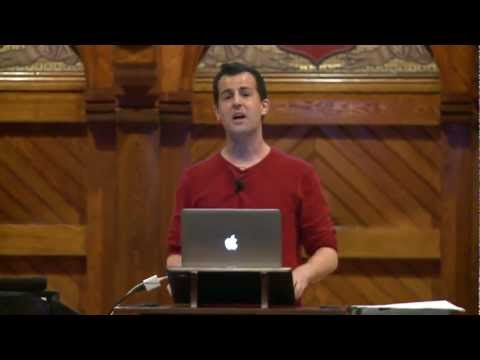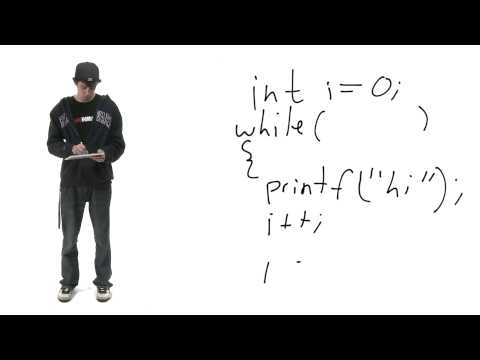# Loops

## Notes

The loop blocks you've used in Scratch can all be recreated in C!

Loops allow for the repetition of code until a condition is met.

There are three types of loops commonly used in C:

• for
• while
• do while

Here's how a for loop works:

Variables are initialized.

The condition is evaluated.

• If the condition is true, the code in the loop's body runs, variables are updated, and the condition is reevaluated.
• If the condition is false, the loop terminates.

This for loop in C is analogous in functionality to the scratch blocks above it.

• i is initialized to 0.
• "This is CS50!" prints.
• i increments.
• The loop terminates when i is equal to 10, and "This is CS50!" will be printed a total of 10 times.

This for loop iterates through the length of a string, capitalizing all alphabetic characters.

Note how we've initialized two variables, i and j, through the use of a comma.

Here's how a while loop works:

The condition is evaluated.

• If the condition is true, the code in the loop's body runs, and the condition is reevaluated.
• If the condition is false, the loop terminates.

This while loop in C is analogous in functionality to the scratch blocks on the left.

• The condition is evaluated.
• count will be printed and decremented.
• The loop terminates when count < 0, and the numbers 10 through 0 have been printed out in descending order.

This while loop iterates through a string, counting each character until the null terminator (\0) is reached.

Here's how a do while loop works:

The code in the loop's body runs once.

The condition is evaluated.

• If the condition is true, the code in the loop's body runs again, and the condition is reevaluated.
• If the condition is false, the loop terminates.

Do while loops are ideal for vetting user input.

• The user is prompted to enter a positive number.
• If the number is less than 1, the user will be reprompted to enter a positive number.## Slides ( / )## While Loop Conversion

Rewrite the following while loop as a do while loop:

``````int n = 0;
while (n <= 0)
{
printf("Please enter a positive integer: ");
n = GetInt();
}``````

Try out some pseudocode here!
```#include <cs50.h>
#include <stdio.h>

int main(int argc, string argv[])
{
// TODO
}
```

## Square

Take as input a non-negative integer, n, no larger than 23. Use nested for loops to print an n-by-n square using hashes (#) at the command line.

``````jharvard@run.cs50.net (~): ./a.out
Give me a number between 1 and 23: 3
###
###
###``````

Try out some pseudocode here!
```#include <cs50.h>
#include <stdio.h>

int main(int argc, string argv[])
{
// TODO
}
```

## Multiples

Prerequisites:

Take as input a number between 1 and 100. Use a for loop to print every multiple of that number between 1 and 100 (inclusive).

``````jharvard@run.cs50.net (~): ./a.out
Give me a number between 1 and 100: 5
5, 10, 15, 20, 25, 30, 35, 40, 45, 50, 55, 60, 65, 70, 75, 80, 85, 90, 95, 100``````

Try out some pseudocode here!
```#include <cs50.h>
#include <stdio.h>

int main(int argc, string argv[])
{
// TODO
}
```

## Myspace

Prerequisites:

Write a program that prompts the user for a message, and then outputs the message with its first letter capitalized, with all letters in alternating case, as per the sample output below. For simplicity, you may assume that the user will only input lowercase letters and spaces.

``````jharvard@run.cs50.net (~): ./a.out

Try out some pseudocode here!
```#include <cs50.h>
#include <ctype.h>
#include <stdio.h>
#include <string.h>

int main (int argc, string argv[])
{

// TODO

}
```

## For Loop Conversion

Prerequisites:

Rewrite the following double `for` loop as a single `for` loop. Make sure it prints exactly the same output, even though you may not have variables `i` and `j` around.

``````for (int i = 0; i < 5; i++)
for (int j = 0; j < 5; j++)
printf("i = %d, j = %d\n", i, j);``````

Try out some pseudocode here!
```#include <cs50.h>
#include <stdio.h>

int main(int argc, string argv[])
{
// TODO
}
```

## Videos#### Monday, Week 1

An introduction to loops#### Tommy's Loops Short

Tommy gives a general overview